3. Parallel derivatives and Christoffel tensors

We begin by remembering that each basis has its own parallel derivative

In:=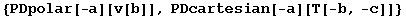Out=defined so that

In:=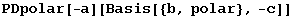Out=This derivative is always flat, and has a vanishing Riemann

In:=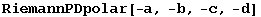Out=But it has torsion, unless the basis is coordinated,

In:=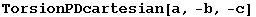Out=In:=Out=In xTensor` we can define the Christoffel tensor connecting two covariant derivatives (cf. Section 6 of xTensorDoc.nb). In particular, this works with the PD of our bases

In:=Out=Notice how the Christoffel connecting any covariant derivative to PD is defined with the derivative, in our case by DefBasis.

In:=Out=Any derivative of any Basis object can be translated into a component of  Christoffel tensors relating that derivative to the PDs of the bases involved

In:=Out=In:=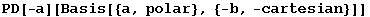Out=In:=Out=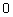With another parallel derivative,

In:=Out=In:=Out=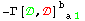Complex bases have FRiemann and AChristoffel tensors, as explained in xTensorDoc, section 6.8.

In:=Global`AChristoffelPDcomp

 Dagger[AChristoffelPDcomp]^=AChristoffelPDcomp† DependenciesOfTensor[AChristoffelPDcomp]^={M3} Info[AChristoffelPDcomp]^={nonsymmetric AChristoffel tensor ,} MasterOf[AChristoffelPDcomp]^=PDcomp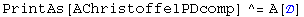ServantsOf[AChristoffelPDcomp]^={AChristoffelPDcomp†} SlotsOfTensor[AChristoffelPDcomp]^={InnerC,-TangentM3,-InnerC} SymmetryGroupOfTensor[AChristoffelPDcomp]^=StrongGenSet[{},GenSet[]] TensorID[AChristoffelPDcomp]^={AChristoffel,PDcomp,PD} xTensorQ[AChristoffelPDcomp]^=True

The FRiemann is also zero

In:=Out=Derivatives of complex bases are also replaced by the corresponding Christoffels

In:=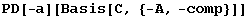Out=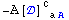In:=Out=In:=Out=In:=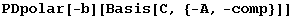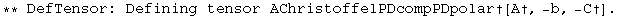Out=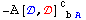Both basis indices must belong to the same vbundle:

In:=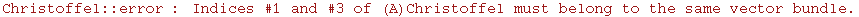Created by Mathematica  (May 16, 2008)